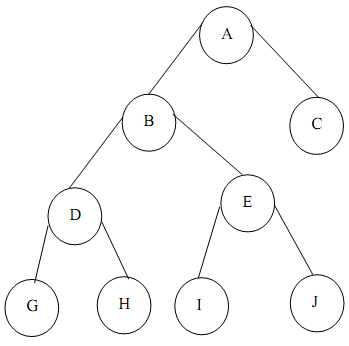## Array-based representation of a binary tree, Data Structure & Algorithms

Assignment Help:

Assume a complete binary tree T with n nodes where each node has an item (value). Label the nodes of the complete binary tree T from top to bottom & from left to right 0, 1, ..., n-1. Relate with T the array A where the ith   entry of A is the item in the node labeled i of T, i = 0, 1, ..., n-1. Table illustrates the array representation of a Binary tree of FigureGiven the index i of a node, we can efficiently & easily compute the index of its parent and left & right children:

Index of Parent: (i - 1)/2, Index of Left Child: 2i + 1, Index of Right Child: 2i + 2.

 Node # Item Left child Right child 0 A 1 2 1 B 3 4 2 C -1 -1 3 D 5 6 4 E 7 8 5 G -1 -1 6 H -1 -1 7 I -1 -1 8 J -1 -1 9 ? ? ?

Table: Array Representation of a Binary Tree

First column illustrates index of node, second column contain the item stored into the node & third & fourth columns mention the positions of left & right children

(-1 shows that there is no child to that specific node.)

#### Algorithm to build a binary tree , Q. Give the algorithm to build a binary ...

Q. Give the algorithm to build a binary tree where the yields of preorder and post order traversal are given to us.

#### State in detail about the integer, State in detail about the Integer ...

State in detail about the Integer Carrier set of the Integer ADT is the set {..., -2, -1, 0, 1, 2, ...}, and  operations on these values are addition, multiplication, subtrac

#### Program of insertion of an element in list, Program will demonstrate the in...

Program will demonstrate the insertion of an element at desired position /* Inserting an element into contiguous list (Linear Array) at particular position */ /* contiguous_

#### User-specified memory location, You need to implement a function which will...

You need to implement a function which will write out a given user-specified memory location to disk in base 10. That means that you have to convert the large number data structure

#### Write an algorithm insert, Q. Write an algorithm INSERT which takes a point...

Q. Write an algorithm INSERT which takes a pointer to a sorted list and a pointer to a node and inserts the node into its correct position or place in the list.  Ans: /* s

#### Calculation of storage complexity, Since memory is becoming more & cheaper,...

Since memory is becoming more & cheaper, the prominence of runtime complexity is enhancing. However, it is very much significant to analyses the amount of memory utilized by a prog

#### Postorder traversal of a binary tree, Postorder traversal of a binary tree ...

Postorder traversal of a binary tree struct NODE { struct NODE *left; int value;     /* can take any data type */ struct NODE *right; }; postorder(struct NODE

#### Find a minimum cost spanning arborescence rooted, Find a minimum cost spann...

Find a minimum cost spanning arborescence rooted at r for the digraph shown below, using the final algorithm shown in class. Please show your work, and also give a final diagram wh

#### The space - time trade off, The Space - Time Trade Off The best algorit...

The Space - Time Trade Off The best algorithm to solve a given problem is one that needs less space in memory and takes less time to complete its implementation. But in practic

#### Ways to implement abstract data types, Ways to implement abstract data type...

Ways to implement abstract data types A large part of the study of data structures and algorithms is learning about alternative ways to implement an ADT and evaluating alternat